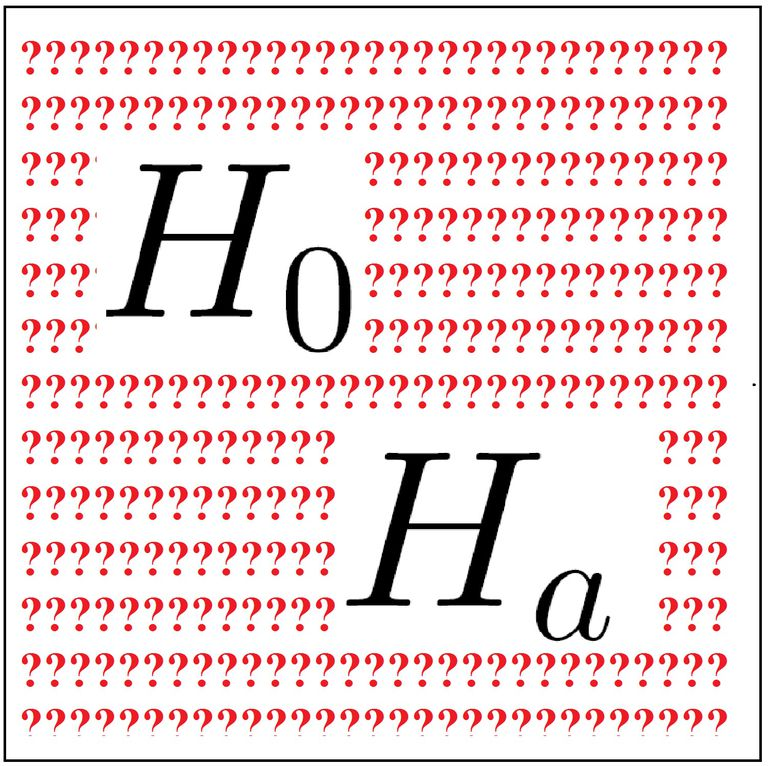# Null Hypothesis

## What is a Null Hypothesis?

A null hypothesis is an initial statement claiming that there is no relationship between two measured events. A null hypothesis is a foundation of the scientific method, as scientists use experiments to accept or reject a null hypothesis based upon the relationship, or lack thereof, between two phenomena. Scientists model the results of the phenomena using what is called a "distribution under a null hypothesis." Often, the null hypothesis is considered to be true until evidence suggests otherwise. A null hypothesis is denoted H0, while an alternative hypothesis is denoted Ha.Source

### Types of Hypotheses

There are several forms of hypotheses used in different contexts to describe varying levels of specificity of an outcome. For example, an exact hypothesis specifies an exact parameter for the hypothesis (e.g. 50), whereas an inexact hypothesis specifies a range (e.g. μ ≤ 60). Additionally, there is what is known as a one-tailed hypothesis. These are inexact hypotheses in which the value of the parameters are defined as either above or equal to a certain value, or below or equal to a certain value. Accordingly, a one-tailed hypothesis is said to have directionality, as a range extends above, or below, a value.

## How does a Null Hypothesis work?

A null hypothesis is used in hypothesis testing, or the testing of predictions about a particular outcome. Hypothesis testing works by collecting data and measuring how likely the particular set of data is when the study is on a randomly selected representative sample. If the data sample is very unlikely relative to the null hypothesis, then the null hypothesis is probably false. If the data do not contradict the null hypothesis, the null hypothesis could be either true or false, depending on interpretation of the data. In that case, either the connection between phenomena is very weak, or there is simply no connection at all.

### Two Types of Null Hypothesis Testing

There are considered to be two methods for null hypothesis testing. The first is the significance testing approach, in which the null hypothesis is rejected if the resulting data is significantly unlikely to have occurred if the null hypothesis were true. In essence with this approach, only with significant evidence indicating otherwise are null hypothesis rejected. The other approach to testing the null hypothesis is through contrast. The null hypothesis and the alternative hypotheses are compared, and the differences in the data help inform and construct the scientist's understandings of the phenomena.